Data Structures and Algorithms with Object-Oriented Design Patterns in C### Conventions for Writing Big Oh Expressions

Certain conventions have evolved which concern how big oh expressions are normally written:

• First, it is common practice when writing big oh expressions to drop all but the most significant terms. Thus, instead of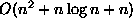we simply write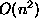.
• Second, it is common practice to drop constant coefficients. Thus, instead of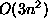, we simply write. As a special case of this rule, if the function is a constant, instead of, say O(1024), we simply write O(1).

Of course, in order for a particular big oh expression to be the most useful, we prefer to find a tight asymptotic bound (see Definition). For example, while it is not wrong to write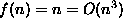, we prefer to write f(n)=O(n), which is a tight bound.

Certain big oh expressions occur so frequently that they are given names. Tablelists some of the commonly occurring big oh expressions and the usual name given to each of them.

 expression name O(1) constant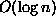logarithmic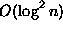log squared O(n) linear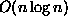n log nquadratic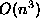cubic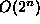exponentialCopyright © 2001 by Bruno R. Preiss, P.Eng. All rights reserved.